>  What is area roughness?  >  Area Roughness Parameters

# Sal (Auto-Correlation Length) | Area Roughness Parameters

## surface texture parameters in ISO 25178

• Height
• Spatial
• Hybrid
• Functional
• Functional volume
• Feature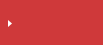Search from the parameters list

## Sal (Auto-Correlation Length)

Sal represents the horizontal distance in the direction in which the auto-correlation function decays to the value[s] (0.2 by default) the fastest.

Str is a measure of uniformity of the surface texture. The value is obtained by dividing the horizontal distance in the direction in which the auto-correlation function decays to the value[s] (0.2 by default) the fastest (equivalent to Sal) by the horizontal distance in the direction of the slowest decay of auto-correlation function to the value[s].

## Str (Texture aspect ratio)

Str is a measure of uniformity of the surface texture. The value is obtained by dividing the horizontal distance in the direction in which the auto-correlation function decays to the value[s] (0.2 by default) the fastest (equivalent to Sal) by the horizontal distance in the direction of the slowest decay of auto-correlation function to the value[s].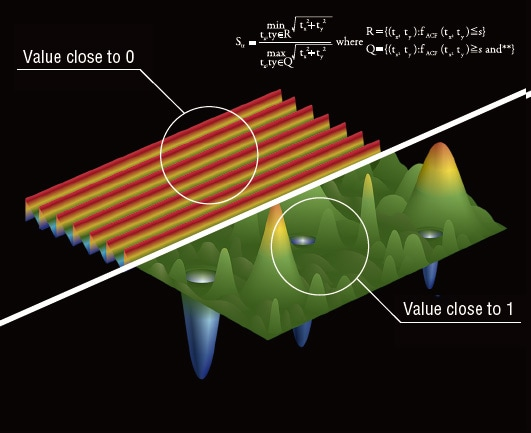Auto-correlation function

In image processing, an auto-correlation function is a measure of the matching ratio between an image rendered in different coordinates and the original image.

The auto-correlation function used for the calculation of Sal and Str is as follows: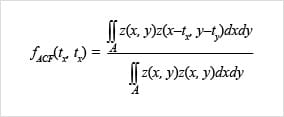The image below explains this function in the X direction for simplicity.

Suppose that there is a height difference, as shown in the data below.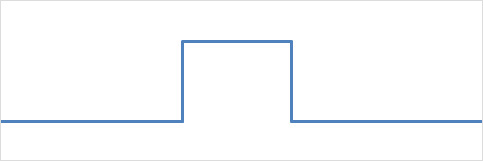The auto-correlation of this data is represented by the following shape: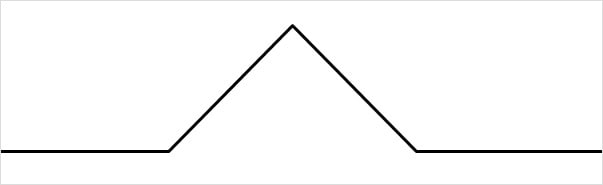The auto-correlation function determines the overlapping area of the data of different height data and the original height data, as shown in the yellow areas in the figure below.

When the difference is small, the overlapping area is large, as is the auto-correlation value.

When the difference is large, the overlapping area is small, as is the auto-correlation value.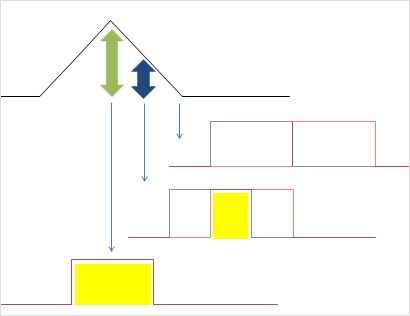A steep height difference decreases an auto-correlatoin immediately because even a small difference causes a great change in the shape; conversely, a gradual height difference decreases an auto-correlation slowly because the shape does not change until the difference becomes large.

Since Sal determines the distance at which the auto-correlation decreases the fastest, it can be used to determine whether there is a point at which the surface height changes abruptly; Str determines the ratio of distances at which the auto-correlation decreases the fastest and slowest, so it can be used to determine the presence of lay.## Search from the parameters list

Height Sa (arithmetical mean height) Sz (Maximum height) Sq (Root mean square height) Ssk (Skewness) Sku (Kurtosis) Sp (Maximum peak height) Sv (Maximum pit height) Sal (Auto-correlation length) / Str (Texture aspect ratio) Std* (Texture direction) Sdq (Root mean square gradient) Sdr (Developed interfacial area ratio) Smr(c) Areal material (bearing area) ratio Smc(mr) Inverse areal material ratio Sk(Core height) Spk (Reduced peak height) Svk (reduced dale height (reduced valley depth)) Smr (Peak material portion) Smr2 (Valley material portion) Sxp (Peak extreme height) Vvv (Dale void volume) Vvc (Core void volume) Vmp (Peak material volume) Vmc (Core material volume) Spd (Density of peaks) Spc (Arithmetic mean peak curvature) S10z / S5p / S5v / Sda(c) / Sha(c) / Sdv(c) / Shv(c)